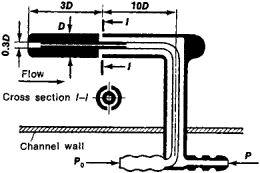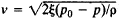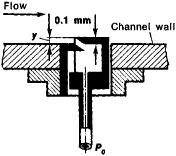# Flow-Measuring Tube

The following article is from The Great Soviet Encyclopedia (1979). It might be outdated or ideologically biased.

## Tube, Flow-Measuring

(Russian, gidrometricheskaia trubka), an instrument for determining the magnitude and direction of the velocity of a fluid flow or the flow rate of a liquid or gas on the basis of the measurement of pressure in the flow. Flow-measuring tubes are used to measure the flow velocities of water in rivers, channels, flumes, and pipes and the relative speeds of ships and aircraft.Figure 1. Diagram of a Pitot-Prandtl tube

The Pitot-Prandtl tube is a widely used flow-measuring tube. It is a cylindrical tube with a hemispherical nose (Figure 1), and its axis is aligned with the flow. The total pressure p0 is measured through a central hole on the hemisphere (the stagnation point); the static pressure p is measured by using one or more other holes I located on the side of the cylinder at a distance of a few tube diameters from the nose and the support. The geometric shape of the tube, the shape of the holes, and the distance from the holes to the nose of the tube are selected so that the pressure in the side holes differs as little as possible from the static pressure at the point under study in the flow. The small pressure discrepancy is taken into account by a correction factor ξ which is determined by calibration. Knowing; p and p0, we calculate the flow velocity v on the basis of Bernoulli’s equation. For an incompressible fluid; the density ρ can be found by using the Clapeyron equation (that is, the equation of state of an ideal gas) or by some other means. When air velocities greater than 50–60 m/-sec are measured, the compressibility of the air must be taken into account. The Pitot-Prandtl tube is also used to determine v and the Mach number M in a supersonic flow.

At low flow velocities (v < 6 m/sec) or in highly rarefied flows, where the Reynolds number Re < 300, a substantial increase in the factor ξ is observed. The Pitot-Prandtl tube may also be used at very low Re, including the case of a free molecule flow (when M/Re> 1), but its practical application for such flows encounters a number of difficulties associated with calibration and the measurement of very low absolute pressures.Figure 2. Diagram of a Stanton tube

For measuring flow velocity, there are numerous modifications of the Pitot-Prandtl tube, such as the Losievskii and Preston tubes; velocity is also measured with the venturi tube. The direction of flow is measured with cylindrical and spherical probes, combinations of three Pitot tubes mounted at an angle to one another, and other instruments whose readings are highly sensitive to the direction of flow.

The Stanton tube, which measures the velocity head in a flow with a large vertical velocity gradient (Figure 2), is used to study velocity fields in the boundary layer of a flow of a viscous liquid or gas near a solid wall. This tube is mounted directly on the surface of a body immersed in a flow and is moved vertically by means of a micrometer screw. The pressure measured with the tube is the pressure at some effective distance from the wall, which is determined by calibration. The velocity is calculated from the difference between the total pressure measured with the tube and the static pressure at the channel wall.

### REFERENCES

Fizicheskie izmereniia v gazovoi dinamike i pri gorenii, parts 1–2. Moscow, 1957. (Translated from English.)
Gorlin, S. M., and I. I. Slezinger. Aeromekhanicheskie izmereniia. Moscow, 1964.
Popov, S. G. Izmerenie vozdushnykh potokov. Moscow-Leningrad, 1947.

L. V. KOZLOV